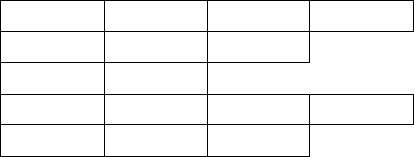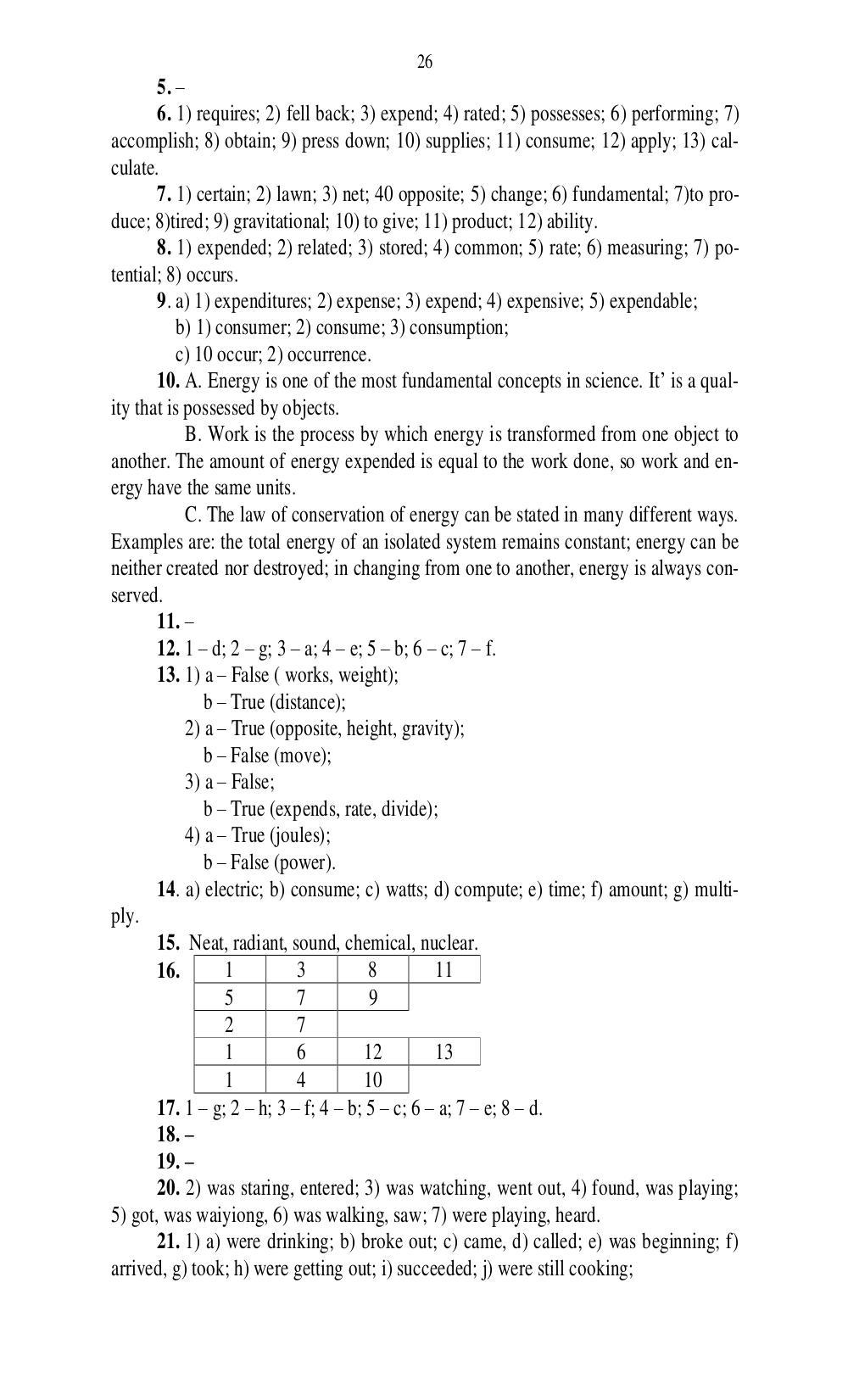# Английский язык. Ч. 1. Ильичева Н.А - 26 стр.

Составители:

Рубрика:

• ## Иностранный язык26
5.
6. 1) requires; 2) fell back; 3) expend; 4) rated; 5) possesses; 6) performing; 7)
accomplish; 8) obtain; 9) press down; 10) supplies; 11) consume; 12) apply; 13) cal-
culate.
7. 1) certain; 2) lawn; 3) net; 40 opposite; 5) change; 6) fundamental; 7)to pro-
duce; 8)tired; 9) gravitational; 10) to give; 11) product; 12) ability.
8. 1) expended; 2) related; 3) stored; 4) common; 5) rate; 6) measuring; 7) po-
tential; 8) occurs.
9. a) 1) expenditures; 2) expense; 3) expend; 4) expensive; 5) expendable;
b) 1) consumer; 2) consume; 3) consumption;
c) 10 occur; 2) occurrence.
10. A. Energy is one of the most fundamental concepts in science. It is a qual-
ity that is possessed by objects.
B. Work is the process by which energy is transformed from one object to
another. The amount of energy expended is equal to the work done, so work and en-
ergy have the same units.
C. The law of conservation of energy can be stated in many different ways.
Examples are: the total energy of an isolated system remains constant; energy can be
neither created nor destroyed; in changing from one to another, energy is always con-
served.
11.
12. 1 d; 2 g; 3 a; 4 e; 5 b; 6 c; 7 f.
13. 1) a False ( works, weight);
b True (distance);
2) a True (opposite, height, gravity);
b False (move);
3) a False;
b True (expends, rate, divide);
4) a True (joules);
b False (power).
14. a) electric; b) consume; c) watts; d) compute; e) time; f) amount; g) multi-
ply.
15. Neat, radiant, sound, chemical, nuclear.
16.
1 3 8 11
5 7 9
2 7
1 6 12 13
1 4 10
17. 1 g; 2 h; 3 f; 4 b; 5 c; 6 a; 7 e; 8 d.
18.
19.
20. 2) was staring, entered; 3) was watching, went out, 4) found, was playing;
5) got, was waiyiong, 6) was walking, saw; 7) were playing, heard.
21. 1) a) were drinking; b) broke out; c) came, d) called; e) was beginning; f)
arrived, g) took; h) were getting out; i) succeeded; j) were still cooking;26
5. –
6. 1) requires; 2) fell back; 3) expend; 4) rated; 5) possesses; 6) performing; 7)
accomplish; 8) obtain; 9) press down; 10) supplies; 11) consume; 12) apply; 13) cal-
culate.
7. 1) certain; 2) lawn; 3) net; 40 opposite; 5) change; 6) fundamental; 7)to pro-
duce; 8)tired; 9) gravitational; 10) to give; 11) product; 12) ability.
8. 1) expended; 2) related; 3) stored; 4) common; 5) rate; 6) measuring; 7) po-
tential; 8) occurs.
9. a) 1) expenditures; 2) expense; 3) expend; 4) expensive; 5) expendable;
b) 1) consumer; 2) consume; 3) consumption;
c) 10 occur; 2) occurrence.
10. A. Energy is one of the most fundamental concepts in science. It’ is a qual-
ity that is possessed by objects.
B. Work is the process by which energy is transformed from one object to
another. The amount of energy expended is equal to the work done, so work and en-
ergy have the same units.
C. The law of conservation of energy can be stated in many different ways.
Examples are: the total energy of an isolated system remains constant; energy can be
neither created nor destroyed; in changing from one to another, energy is always con-
served.
11. –
12. 1 – d; 2 – g; 3 – a; 4 – e; 5 – b; 6 – c; 7 – f.
13. 1) a – False ( works, weight);
b – True (distance);
2) a – True (opposite, height, gravity);
b – False (move);
3) a – False;
b – True (expends, rate, divide);
4) a – True (joules);
b – False (power).
14. a) electric; b) consume; c) watts; d) compute; e) time; f) amount; g) multi-
ply.
15. Neat, radiant, sound, chemical, nuclear.
16.        1         3          8         11
5         7          9
2         7
1         6         12         13
1         4         10
17. 1 – g; 2 – h; 3 – f; 4 – b; 5 – c; 6 – a; 7 – e; 8 – d.
18. –
19. –
20. 2) was staring, entered; 3) was watching, went out, 4) found, was playing;
5) got, was waiyiong, 6) was walking, saw; 7) were playing, heard.
21. 1) a) were drinking; b) broke out; c) came, d) called; e) was beginning; f)
arrived, g) took; h) were getting out; i) succeeded; j) were still cooking;